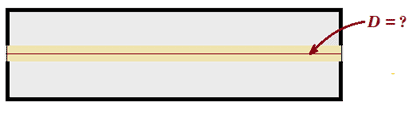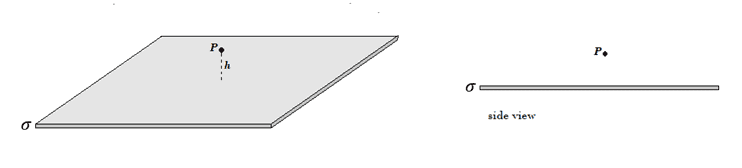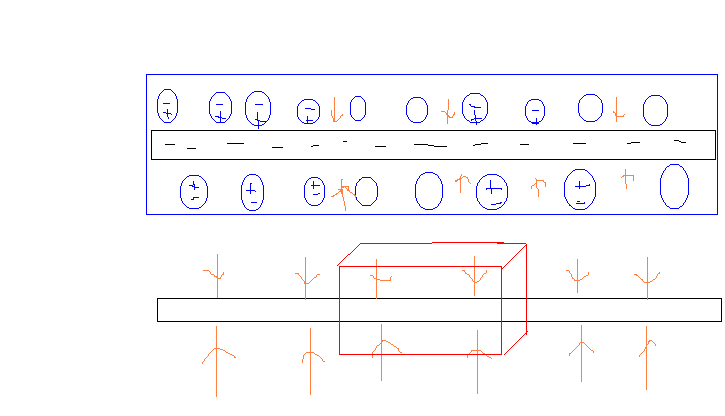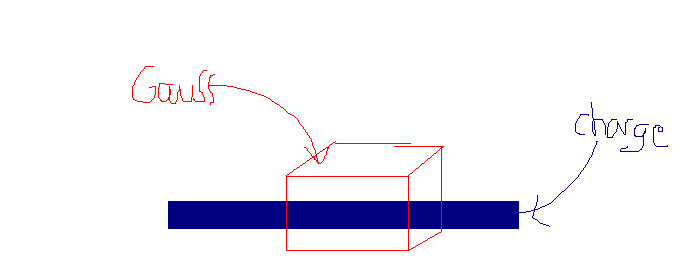# Bound charges of a block (top and bottom surface)

happyparticle
Homework Statement:
Consider a block of surface ##25cm^2## and thickness 12mm made of lucid a dielectric with ##\epsilon_r = 3.2##. We charge the center of this block with a beam of electron 0.1##\mu A## for 1 second to have a uniform charged layer of 2mm of thickness where the center is at 6mm of the surface of the block.
Relevant Equations:
##\sigma p = \vec{P} \cdot \hat{n}##

##\vec{P} = \epsilon_0 \chi_e \vec{E} = \frac{\chi_e \vec{D}}{\epsilon_r}##
From what I think, to find the bound charges of a block on the top and bottom surface I have to find the electric field or the displacement (D).
However, I'm not sure how to proceed with a cube. For example, with a sphere ##E = \frac{Q}{4\pi \epsilon_0 r^2}## since r is constant.

For a cube, it depends where I'm at the surface, since the electric field is produce by the charged layer it the center of the cube, r is not the same everywhere on the surface.
Am I correct?

Homework Helper
Gold Member
My hint would be to use Gauss' law for the field ##\mathbf{D}## in order to find ##\mathbf{D}## at the top and bottom surfaces of the block.

But first, decide what ##\mathbf{D}## must be on the central plane of the block:[EDIT: It is not necessary to know the value of D on the central plane in order to determine D at the top and bottom surfaces. If you do see what D is on the central plane, then you can use a Gaussian surface where part of the Gaussian surface lies on the central plane. Alternately, there is a different choice of Gaussian surface that does not use the central plane but still allows you to obtain D at the upper and lower surfaces.]

Last edited:
happyparticle
I though about that, but I'm not sure how to use it.
I know that ##\vec{E} = \frac{\vec{D}}{\epsilon}##

##\oint_S \vec{D} \cdot \vec{da} = Q##

##\vec{D} \cdot A = 1\cdot 10^{-7} \cdot A##

##\vec{D} = 1\cdot 10^{-7} ##
Is this makes sense?

Last edited by a moderator:
Gordianus
What closed surface did you choose?

happyparticle
Same as the plane, 0.05m x 0.002m

Gordianus
You chose an open surface whereas Gauss' law requieres a closed one.

happyparticle
My bad I wanted to say, ##0.05m^2## x 0.002m like a plane, but I don't know if this is correct either.

Gordianus
I suggest drawing some field lines in red and the closed surface in blue. Now analize the dot product.

happyparticle
I don't understand what's wrong. isn't Q the charges inside the volume and ##\int da## the area of the charged region?
Is ##\vec{D}## the same direction than ##\vec{E}## ?

Gordianus
No. Gauss' law refers to a closed surface that encloses the charge. It isn't the área of the charged region.

happyparticle
So, Gauss' law can only encloses charges on the surface? and the surface could be (0.002m + ##\epsilon##) x( 0.05 + ##\epsilon##), but that doesn't help me, since I don't know the charge on the surface and the surface can be anything.

Gordianus
It's past midnight where I live and I'm tired. I'll be back tomorrow.

happyparticle
All right, thanks for you help.

happyparticle
Following a tutorial. I found something, but I'm not sure if this is correct.
If a choose a Gauss' surface to be a rectangle

##10^{-7}\int_0^L dx = Q ##
##Q = 10^{-7} L ##

##\int_0^L \int_0^l dxdy= 10^{-7} L ##

##D = \frac{10^{-7}}{l}##

I don't understand that D can be anything.

Last edited by a moderator:
Gordianus
I´m back and I insist with a previous idea. Can you draw some field lines in red and the closed surface in blue? (##\vec D## and ##\vec E## point in the same direction)

happyparticle
All right, I did it , but I'm not sure what should I see.
Since the charged part is negatively charged the field point inward.
I don't know what I'm looking for. I guess top = - bottom, I don't know.
I'm using a gaussian pillbox.

Last edited by a moderator:
Gordianus
Now try to "see" ##\oint_S {\vec D \cdot d\vec S}##

Homework Helper
Gold Member
It might be helpful for you to review the standard problem of finding the electric field of an infinite plane sheet with surface charge density ##\sigma##. How would you construct a Gaussian surface to find ##E## at point ##P##?happyparticle
I think cube or cylinder works, but cylinder seems easier?
Now try to "see" ##\oint_S {\vec D \cdot d\vec S}##
##D \hat{y} \cdot A \hat{y} + D (-\hat{y}) \cdot A (-\hat{y}) = Q##

##D = \frac{\sigma}{2}##

I draw something, but I have to do with my mouse so it's ugly. This is how I see the system.Last edited by a moderator:
Homework Helper
Gold Member
##D \hat{y} \cdot A \hat{y} + D (-\hat{y}) \cdot A (-\hat{y}) = Q##

##D = \frac{\sigma}{2}##
Ok.

At what point or points in the system does D have this magnitude?

What are you going to use for ##\sigma##? The electrons are spread throughout a volume rather than spread over a surface.

happyparticle
Ok.

At what point or points in the system does D have this magnitude?

What are you going to use for ##\sigma##? The electrons are spread throughout a volume rather than spread over a surface.
Since ##\sigma## is the charge on a surface, I think at the top and bottom surface of the charged volume D has this magnitude.

The total charge should be ##10^{-7}##

I think what I did doesn't work.

Last edited by a moderator:
Homework Helper
Gold Member
Since ##\sigma## is the charge on a surface, I think at the top and bottom surface of the charged volume D has this magnitude.
Let's make sure that you are understanding the application of Gauss' law.
Apparently, from Gauss' law applied to your pillbox you got
##D \hat{y} \cdot A \hat{y} + D (-\hat{y}) \cdot A (-\hat{y}) = Q##
What does the first term on the left side represent? At what point or points is ##D## being evaluated in this term?

Also, it looks to me like you got the directions for ##\vec D## wrong in the two terms on the left side.

The total charge should be ##10^{-7}##
Is this the total charge in the electron layer? What are the units? What should be the sign of the charge?

If ##10^{-7}## is the total charge then ##\frac{10^{-7}}{A}## is the charge on top and bottom surface.
I don't understand this. The electrons are spread throughout a volume which is a thin slab of area ##A_{slab}## and thickness ##t_{slab}##. The thin slab has a volume charge density ##\rho_{slab}## which you can easily calculate. It doesn't make much sense to talk about "the charge on the top and bottom surface" of the thin slab.

##D \hat{y} \cdot A \hat{y} + D (-\hat{y}) \cdot A (-\hat{y}) = Q##

##Q## is the charge enclosed in your pillbox. You should be able to express this in terms of the area ##A## of the top (or bottom) of your pillbox, ##\rho_{slab}##, and ##t_{slab}##. When you solve the equation for ##D##, you will be able to express ##D## in terms of ##\rho_{slab}## and ##t_{slab}##.

happyparticle
I don't understand this. The electrons are spread throughout a volume which is a thin slab of area Aslab and thickness tslab. The thin slab has a volume charge density ρslab which you can easily calculate. It doesn't make much sense to talk about "the charge on the top and bottom surface" of the thin slab.
I that point I just try anything. I know what I did is wrong, but I don't know what should I do. I found that here https://en.wikipedia.org/wiki/Electric_displacement_field

happyparticle
What does the first term on the left side represent? At what point or points is D being evaluated in this term?
I though it was on the surface. I have no idea otherwise.

##
D (-\hat{y}) \cdot A \hat{y} + D (\hat{y}) \cdot A (-\hat{y}) = Q
##
This should be right.

You said Q is the charge enclosed by the gauss' surface, which make sense because Gauss' law is the integral of surface = integral of volume.

However, I think where I'm really confuse is that Q = all the charge inside the Gauss' surface, thus Q depends of the surface so the result can be different. Or only the part that is charged inside de Gauss' surface, but I don't see how to get it. Basically, that's my issue since the beginning.

Does
##
D (-\hat{y}) \cdot A \hat{y} + D (\hat{y}) \cdot A (-\hat{y}) = Q
##
mean that D on both surface of the pillbox = the total charge inside the volume?
The charge doesn't fill the entire Gauss'surface.
I only have the total charge, ##10^{-7}##All the examples I found they have a plane without thickness.
So ##2DA = \sigma A ##
##D = \frac{\sigma}{2}##
If I have a plane with a thickness I guess
So ##2DA = \sigma A \cdot thickness ##

But again, it depends of the Gauss' surface.

I'm really stuck I don't know.

Last edited by a moderator:
Homework Helper
Gold Member
Does
##
D (-\hat{y}) \cdot A \hat{y} + D (\hat{y}) \cdot A (-\hat{y}) = Q
##
mean that D on both surface of the pillbox = the total charge inside the volume?
It doesn't make sense to say that ##D## equals a charge. ##D## and ##Q## do not have the same dimensions, so they can't be equal.

The equation is stating that the total flux of ##\vec D## through the total surface of the pillbox equals the total "free" charge enclosed by the pillbox. (Flux of ##\vec D## and charge have the same dimensions.)

Only the top and bottom surfaces of the pillbox have a nonzero flux of ##\vec D##. The first term on the left side of your equation represents the flux of ##\vec D## through the top of the pillbox. Note that this term reduces to ##-DA## where ##D## is the magnitude of ##\vec D## at points on the top surface of the pillbox and ##A## is the area of the top of the pillbox. If the pillbox is symmetrically placed relative to the electron layer, then ##D## on the bottom surface of the pillbox has the same value as on the top of the pillbox. So, the flux of ##\vec D## through the bottom surface of the pillbox equals the flux through the top surface. So, overall, the total flux of ##\vec D## through the entire surface of the pillbox is just twice the flux through the top surface of the pillbox. That is, the total flux is ##-2DA##. So, your equation reduces to

##-2DA = Q##

where ##D## is the magnitude of ##\vec D## at the top surface of your pillbox, ##A## is the area of the top surface of the pillbox, and ##Q## is the total charge enclosed by the pillbox. Since ##D## and ##A## are positive quantities, the left side of the equation is a negative quantity. Great, because we know the right-hand side, ##Q##, is also negative as it represents the "free" charge (charge of the electrons) enclosed by the pillbox.

Let ##\rho_f## be the volume charge density of the free electrons in the electron layer. Let ##t## be the thickness of the electron layer. How would you express ##Q## in terms of the symbols ##\rho_f##, ##t##, and the area, ##A##, of the top of the pillbox? Use this expression for ##Q## in the previous equation and solve for ##D##.

•happyparticle
happyparticle
Let ρf be the volume charge density of the free electrons in the electron layer. Let t be the thickness of the electron layer. How would you express Q in terms of the symbols ρf, t, and the area, A, of the top of the pillbox? Use this expression for Q in the previous equation and solve for D.
##Q = \rho_f \cdot t \cdot A ##
If this is correct, is Q alway the total charge of the inside the Gauss' surface.
For a plane without thickness for example, ##Q = \sigma \cdot A## ?

Homework Helper
Gold Member
##Q = \rho_f \cdot t \cdot A ##
If this is correct, is Q alway the total charge of the inside the Gauss' surface.
For a plane without thickness for example, ##Q = \sigma \cdot A## ?
Yes, that’s right.

happyparticle
I could have find the electric field without knowing D?

I found D = ##\frac{\rho \cdot t}{2} = \frac{10^{-7} \cdot 0.002m}{2} = 10^{-10}##
##\rho## is not correct here, I'll try to fix it. ##\rho## is ##c/m^3## and ##10^{-7}## is c.
##E = \frac{D}{\epsilon} = \frac{10^{-10}}{8.854\cdot 10^{-12} \cdot 3.2}##
##V = -\int_a^b E \cdot dl## To find the potential inside the slab, should I get the electric field for both the top and bottom surface then integrate from the surfaces to the center of the slab.

Last edited by a moderator:
Homework Helper
Gold Member
I could have find the electric field without knowing D?
Are you asking if it is possible to find E inside the dielectric without finding D first?

If you are going to use Gauss' law for E to find E, then you would need to know both the free charge and the bound charge inside your Gaussian surface. But the bound charge is an unknown that you are trying to find.

Using Gauss' law for D to find D requires only knowing the free charge inside the Gaussian surface. You can then find E by using the relation between E and D inside the dielectric.

At this point you should know D inside the dielectric except at points inside the electron layer. You should be able to use the equations you listed in the "relevant equations" section of your first post to find the bound charge on the upper and lower surfaces of the dielectric.

Homework Helper
Gold Member
I found D = ##\frac{\rho \cdot t}{2} = \frac{10^{-7} \cdot 0.002m}{2} = 10^{-10}##
You have not used the correct value for the free charge density ##\rho## of the electron layer.
Please include units with the numerical values.

happyparticle
You have not used the correct value for the free charge density ##\rho## of the electron layer.
Please include units with the numerical values.
Yes, I saw that, I edited too late.

All right,
##D = \frac{0.02 c/m^3 \cdot 0.002m}{2} = 0.00002 c/m^2##
##E = 705896 v/m##

Finally, I found ##\sigma = -0.000014 c/m^2## for both surface of the block.

Last edited by a moderator:
Homework Helper
Gold Member
Yes, I saw that, I edited too late.

All right,
##D = \frac{0.02 c/m^3 \cdot 0.002m}{2} = 0.00002 c/m^2##
##E = 705896 v/m##

Finally, I found ##\sigma = -0.000014 c/m^2## for both surface of the block.
Those answers look correct.The values that you give for D and E are good for points inside the dielectric except for points within the electron layer. (Of course, we are using an approximation where we are neglecting any fringing of the fields.)

The use of scientific notation and rounding to an appropriate number of significant figures would be nice.

happyparticle
All right, I have few more questions.
I found E and D using free charges inside the slab. however, since I don't have free charges inside the other part of the dielectric does it means that ##\sigma_f = 0## and ##\rho_f = 0##, even if a dielectric has a polarization density.

Gordianus
Yes. This system has "free" volumen charge only un the central slab

happyparticle
All right, it took me some time to understand, but thank you both for your help. Things all clearer now.

•TSny